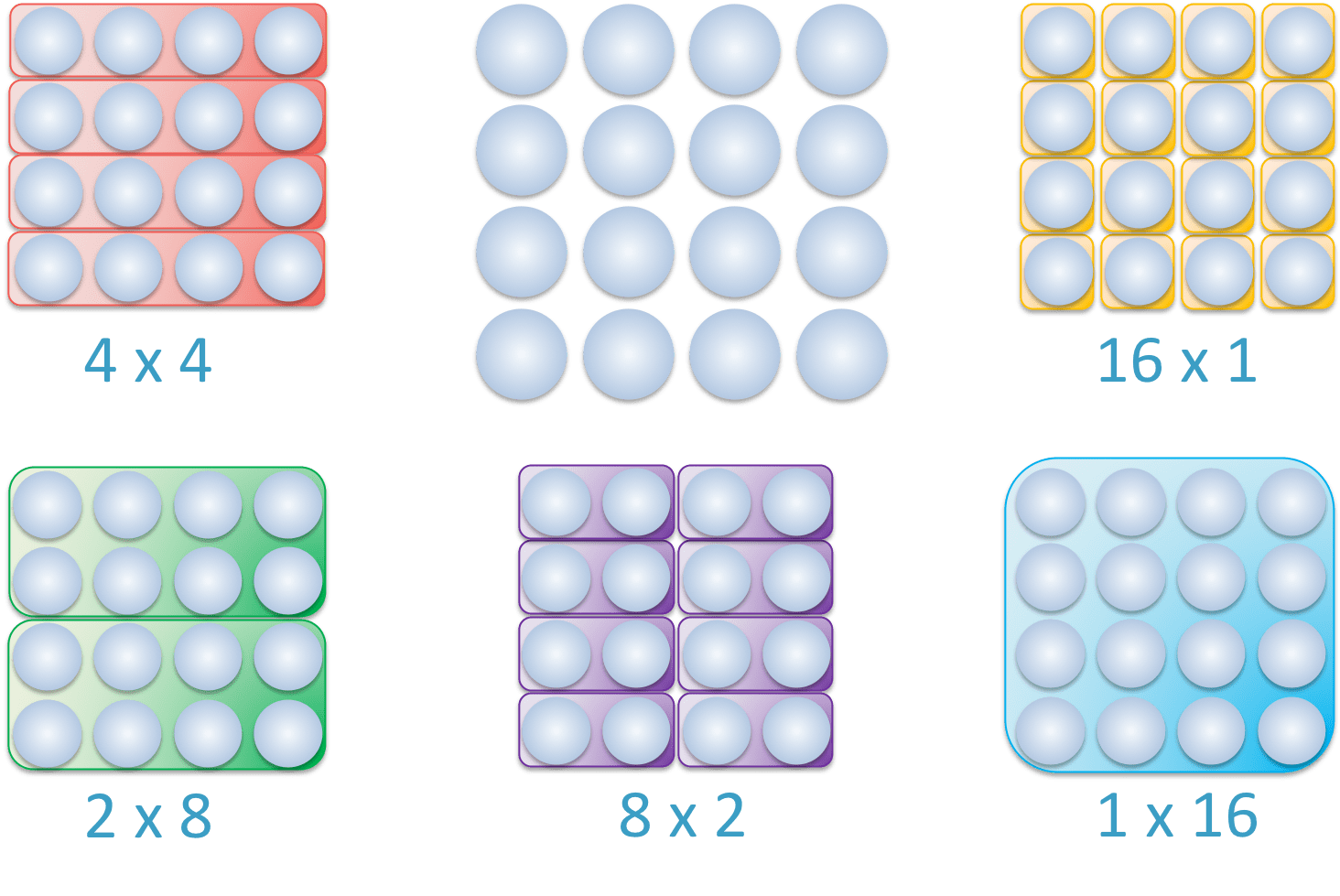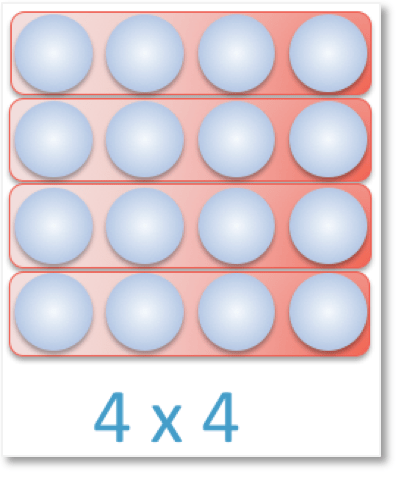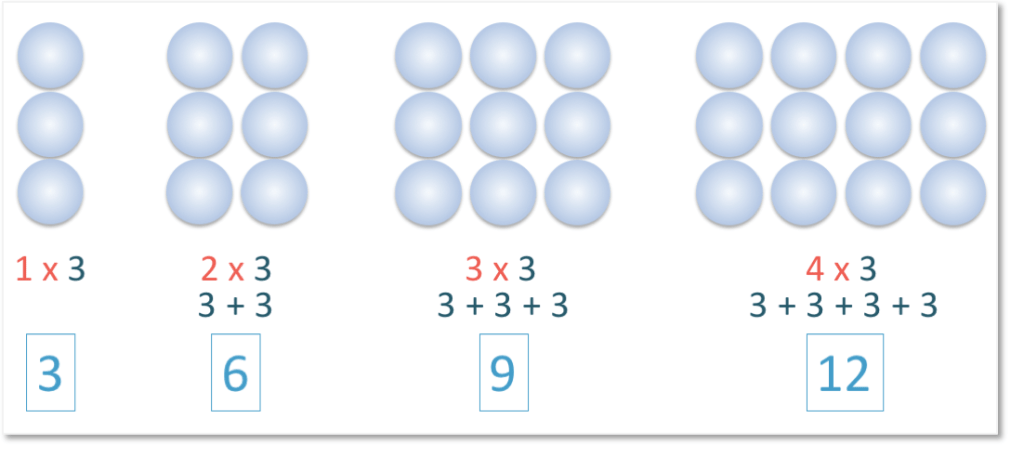# Multiplication as Equal Groups

Multiplication as Equal Groups• Here we have 16 counters arranged in an .
• We can write a for every equal group of items that we have.
• A group is an equal group if it has the same number of items as all of the other groups.
• Multiplication means ‘equal groups of’ and is written with a multiplication sign: ‘×’.
• For example: 4 × 4 can be read as ‘4 equal groups of’ 4.
The amount of groups multiplied by the number of counters in each group form our total.• Here we have 16 counters and we will group together counters that form the number 16.
• We have 1 group of 16, so 1 × 16 = 16.
• We have 2 equal groups of 8 counters, so 2 × 8 = 16.
• We have 4 equal groups of 4 counters, so 4 × 4 = 16.
• We have 8 equal groups of 2 counters, so 8 × 2 = 16.
• We have 16 equal groups of 1 counter, so 16 × 1 = 16.
• The number of groups multiplied by the number of counters in each group form our times tables.Supporting Lessons# Introducing Multiplication by Grouping Objects into Equal Groups

Multiplication by grouping into equal groups is a visual and hands-on approach to introducing multiplication. Equal groups are collections of items formed by sorting the total number of items into piles that have exactly the same number of items in each pile.

Multiplication by grouping equal parts would be one of the first ways of introducing multiplication to a child and should be taught using physical objects, such as counters or food items. We use this method to introduce our

before we memorise them. The idea behind multiplication as grouping is to represent multiplication as collecting equal groups of an amount.

In our first example of multiplication by grouping, we have 16 counters:

When teaching multiplication by grouping, you should count the total number first with your child by counting the items individually.We will look at all of the different ways in which we can group the total amount of 16 into equal portions.We can have 4 equal groups, with each containing exactly 4 counters.

This can be written as 4 ‘equal groups of’ 4.

We can rewrite this in maths with multiplication.

The multiplication sign: ‘x’, means ‘lots of’ or ‘equal groups of’.

We can write 4 ‘equal groups of’ 4 as: 4 x 4.

Here is another example of a multiplication group that makes 16:We have 2 lots of 8.

We have two groups, each containing exactly 8 counters. So again we have equal groups.

We can write this as 2 x 8.We can have 8 equal groups of 2, which we can write as 8 x 2.If we look at the entire collection of 16 counters as one group we can have 1 group of 16.

We can write this as 1 x 16.Finally, we can have 16 groups of 1.

Again, all of the 16 parts contain an equal amount of counters. There is 1 counter in every group.

We can write this as 16 x 1.Above is a summary of the five different ways that we can put 16 counters into equal groups of the same number. This shows the different ways that we can multiply numbers to equal 16.

We have formed these multiplication groups by dividing the total amount into equal parts, however when teaching multiplication as grouping, you may want to separate the groups of counters out.

The main concept to teach here is that we already know the total number of counters and therefore we already know the answer to our multiplication.

We count the number of counters in a group and because we know they are divided into equal groups, we know that there are the same number of counters in all of the groups.

We just need to count the total number of groups to create our times table multiplication sum.

Use the ‘multiplication as equal groups’ worksheets above to practice this concept with your child, by circling equal groups of counters.Now try our lesson on ‘What are Multiplication Arrays?‘ where we learn what multiplication arrays are and how to use arrays to teach times tables.